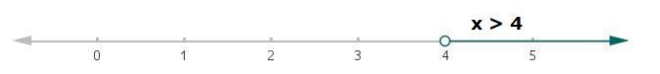# Solve each of the following in equations and represent the solution set onQuestion:

Solve each of the following in equations and represent the solution set on the number line.

$\frac{x}{4}<\frac{(5 x-2)}{3}-\frac{(7 x-3)}{5}$ where $x \in \mathbf{R}$.

Solution:

Given:

$\frac{x}{4}<\frac{(5 x-2)}{3}-\frac{(7 x-3)}{5}$, where $x \in R$

Multiplying 60 on both the sides in the above equation,

$\frac{x}{4}(60)<\frac{(5 x-2)}{3}(60)-\frac{(7 x-3)}{5}(60)$

$15 x<20(5 x-2)-12(7 x-3)$

$15 x<100 x-40-84 x+36$

$15 x<16 x-4$

Now, subtracting 16x from both sides in above equation

$15 x-16 x<16 x-4-16 x$

$-x<-4$

Now, multiplying by -1 on both sides in above equation

$(-x)(-1)<(-4)(-1)$

$x>4$ (inequality sign reversed)